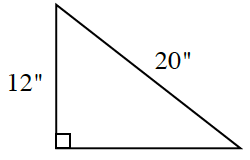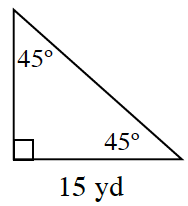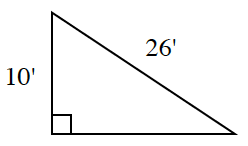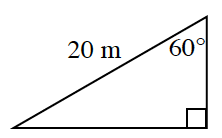### Home > INT2 > Chapter 6 > Lesson 6.1.2 > Problem6-19

6-19.

Use the patterns you have investigated to determine the missing side lengths of each of the triangles below. Do not use a calculator.1.$12$ and $20$ are both multiples of $4$.

1.This triangle is half of a square.

1.$24$ feet

1.This triangle is half of an isosceles triangle.

The short leg is $10$ m.